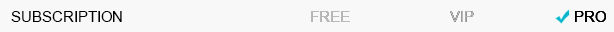# OutdoorsHave more questions? Submit a request

<% if (previousArticle || nextArticle) { %>
<% if (previousArticle) { %>
<% if (previousTitle) { %>

#### <%= previousTitle %>

<% } %> <%= previousArticle.title %>
<% } %> <% if (nextArticle) { %>
<% if (nextTitle) { %>

#### <%= nextTitle %>

<% } %> <%= nextArticle.title %>
<% } %>
<% } %>
<% if (items.length > 1) { %>

<% items.forEach(function(item) { %>
1. <%= item.name %> <%= partial('partial-table-of-contents', { items: item.children, partial: partial }) %>
2. <% }); %>
<% } %>
<% if (items.length) { %>
<% items.forEach(function(item) { %>
1. <%= item.name %> <%= partial('partial-table-of-contents', { items: item.children, partial: partial }) %>
2. <% }); %>
<% } %>
<% var getColumnClasses = function(columnNumber) { var classNames = numberColumns === 'auto' ? 'col-auto' : 'col-12'; if (numberColumns >= 2) classNames += ' md:col-6'; if (numberColumns >= 3) classNames += ' lg:col-4'; if (numberColumns >= 4) classNames += ' xl:col-3'; return classNames; } %> <% var promotedArticlesAbove = 'none' !== 'none' && 'after' === 'before'; %> <% var notificationAbove = 'none' === 'home' && !!'notification_content' && !window.sessionStorage.getItem('alpine:notification:dismissed'); %>
<% var maxSections = 5 %>
<% if (sections.length) { %>
<% sections.forEach(function(section) { %>
• <%= partial('partial-article-list-sections', { id: 'section-' + section.id, parentId: '#' + id, sections: section.sections, activeCategoryId: activeCategoryId, activeSectionId: activeSectionId, activeArticleId: activeArticleId, partial: partial }) %> <% if (section.articles.length) { %> <% } %>
• <% }); %>
<% } %>
<% if (sections.length) { %>
<% sections.slice(0, maxSections).forEach(function(section) { %>
• <%= section.name %> <%= partial('partial-section-list-sections', { parent: section, sections: section.sections, maxSections: maxSections, partial: partial }) %>
• <% }); %> <% if (sections.length > maxSections) { %>
• See more
• <% } %>
<% } %>